NEET  >  31 Year NEET Previous Year Questions: Thermal Properties of Matter

# 31 Year NEET Previous Year Questions: Thermal Properties of Matter

Test Description

## 39 Questions MCQ Test Physics Class 11 | 31 Year NEET Previous Year Questions: Thermal Properties of Matter

31 Year NEET Previous Year Questions: Thermal Properties of Matter for NEET 2023 is part of Physics Class 11 preparation. The 31 Year NEET Previous Year Questions: Thermal Properties of Matter questions and answers have been prepared according to the NEET exam syllabus.The 31 Year NEET Previous Year Questions: Thermal Properties of Matter MCQs are made for NEET 2023 Exam. Find important definitions, questions, notes, meanings, examples, exercises, MCQs and online tests for 31 Year NEET Previous Year Questions: Thermal Properties of Matter below.
Solutions of 31 Year NEET Previous Year Questions: Thermal Properties of Matter questions in English are available as part of our Physics Class 11 for NEET & 31 Year NEET Previous Year Questions: Thermal Properties of Matter solutions in Hindi for Physics Class 11 course. Download more important topics, notes, lectures and mock test series for NEET Exam by signing up for free. Attempt 31 Year NEET Previous Year Questions: Thermal Properties of Matter | 39 questions in 80 minutes | Mock test for NEET preparation | Free important questions MCQ to study Physics Class 11 for NEET Exam | Download free PDF with solutions
 1 Crore+ students have signed up on EduRev. Have you?
31 Year NEET Previous Year Questions: Thermal Properties of Matter - Question 1

### N molecules each of mass m of a gas  A and 2N molecules each of mass 2m of gas B are contained in the same vessel which is maintained at temperature T. The mean square velocity of molecules of B type is v2 and the mean square rectangular component of the velocity of A type is denoted by ω2. Then ω2/v2 

Detailed Solution for 31 Year NEET Previous Year Questions: Thermal Properties of Matter - Question 1

Mean kinetic energy of the two types of molecules should be equal. The mean square velocity of A type molecules = ω2 + ω2 + ω2 = 3ω2

Therefore,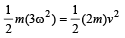This gives ω2 / v2 = 2/3

31 Year NEET Previous Year Questions: Thermal Properties of Matter - Question 2

### 10 gm of  ice  cubes at 0°C are released in a tumbler (water equivalent 55 g) at 40°C.Assuming that negligible heat is taken from the surroundings, the temperature of water in the tumbler becomes nearly (L = 80 cal/g) 

Detailed Solution for 31 Year NEET Previous Year Questions: Thermal Properties of Matter - Question 2

Let the final temperature  be T Heat gained by ice  = mL + m × s × (T – 0) = 10 × 80 + 10 × 1 × T
Heat lost by water = 55 × 1× (40 – T)
By using law of calorimetery, 800 + 10 T = 55 × (40 – T)
⇒ T = 21.54°C = 22°C

31 Year NEET Previous Year Questions: Thermal Properties of Matter - Question 3

### A centigrade and a Fahrenheit thermometer are dipped in boiling water. The water temperature is lowered until the Fahrenheit thermometer registers 140°. What is the fall in temperature as registered by the Centigrade thermometer ?

Detailed Solution for 31 Year NEET Previous Year Questions: Thermal Properties of Matter - Question 3

Using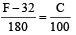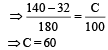Temperature of boiling water = 100°C
We get, fall in temperature = 100 – 60 = 40°C

31 Year NEET Previous Year Questions: Thermal Properties of Matter - Question 4

Thermal capacity of 40 g of aluminium (s = 0.2 cal /g K) is 

Detailed Solution for 31 Year NEET Previous Year Questions: Thermal Properties of Matter - Question 4

Thermal capacity = ms = 40 × 0.2 = 8 cal/°C = 4.2 × 8 J = 33.6 joules/°C

31 Year NEET Previous Year Questions: Thermal Properties of Matter - Question 5

Mercury thermometer can be used to measure temperature upto 

Detailed Solution for 31 Year NEET Previous Year Questions: Thermal Properties of Matter - Question 5

Mercury thermometer is based on the principle of change of volume with rise of temperature and can measure temperatures ranging from –30°C to 357°C.

31 Year NEET Previous Year Questions: Thermal Properties of Matter - Question 6

If the temperature of the sun is doubled, the rate of energy  received on earth will be increased by a factor of 

Detailed Solution for 31 Year NEET Previous Year Questions: Thermal Properties of Matter - Question 6

Amount of energy radiated ∝ T4

31 Year NEET Previous Year Questions: Thermal Properties of Matter - Question 7

A body cools from 50.0°C to 48°C in 5s. How long will it take to cool from 40.0°C to 39°C?Assume the temperature of surroundings to be 30.0°C and Newton's law of cooling to be valid.

Detailed Solution for 31 Year NEET Previous Year Questions: Thermal Properties of Matter - Question 7

Rate of cooling ∝ temperature difference
between system and surrounding.
As the temperature difference is halved, so the rate of cooling will also be halved.
So time taken will be doubled
t = 2 × 5 sec. = 10 sec.

31 Year NEET Previous Year Questions: Thermal Properties of Matter - Question 8

A beaker full of hot water is kept in a room. If it cools from 80°C to 75°C in t1 minutes, from 75° C to 70°C in t2 minutes and from 70°C to 65°C in t3 minutes, then 

Detailed Solution for 31 Year NEET Previous Year Questions: Thermal Properties of Matter - Question 8

Let θ0 be the temperature of the surrounding.
Then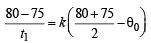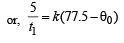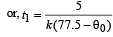… (1)

Similarly,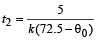…(2)

and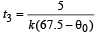… (3)
From (1), (2) & (3), it is obvious that

t1 < t2 < t3

31 Year NEET Previous Year Questions: Thermal Properties of Matter - Question 9

A black body is at temperature of 500 K. It emits energy at rate which is proportional to 

Detailed Solution for 31 Year NEET Previous Year Questions: Thermal Properties of Matter - Question 9

According to Stefan's Law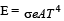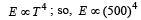31 Year NEET Previous Year Questions: Thermal Properties of Matter - Question 10

The radiant energy from the sun, incident normally at the surface of earth is 20 k cal/m2 min. What would have been the radiant energy, incident normally on the earth, if the sun had a temperature, twice of the present one? 

Detailed Solution for 31 Year NEET Previous Year Questions: Thermal Properties of Matter - Question 10

According to Stefan’s law

E ∝ T4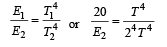⇒ E2 = 320 kcal/m2. min.

31 Year NEET Previous Year Questions: Thermal Properties of Matter - Question 11

If 1 g of steam is mixed with 1 g of ice, then the resultant temperature of the mixture is 

Detailed Solution for 31 Year NEET Previous Year Questions: Thermal Properties of Matter - Question 11

Heat required by ice a t 0° C to reach a temperature of 100°C = mL + mcΔθ = 1 × 80 + 1 × 1 × (100 – 0) = 180 cal
Heat available with 1 g steam to condense into 1 g of water at 100°C = 536 cal.
Obviously the whole steam will not be condensed and ice will attain a temperature of 100°C; so the temperature of mixture = 100°C.

31 Year NEET Previous Year Questions: Thermal Properties of Matter - Question 12

The coefficient of linear expansions of brass and steel are α1 and α2 respectively. When we take a brass rod of length ℓ1 and a steel rod of length ℓ2 at 0ºC, then the difference in their lengths (ℓ2 – ℓ1) will remain the same at all temperatures if [1995, 1999]

Detailed Solution for 31 Year NEET Previous Year Questions: Thermal Properties of Matter - Question 12

Let ΔT be increase in the temperature of brass wire.Then length of brass wire,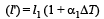Similarly, length of steel wire when temperature is increased by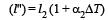According to question , l' – l'' = l1 – l2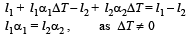31 Year NEET Previous Year Questions: Thermal Properties of Matter - Question 13

The presence of gravitational field is required for the heat transfer by 

Detailed Solution for 31 Year NEET Previous Year Questions: Thermal Properties of Matter - Question 13

In convection, the temperature gradient exists in the vertical direction and not in the horizontal direction. So, up and down movement of particles takes place which depends on the weight and gravity.

31 Year NEET Previous Year Questions: Thermal Properties of Matter - Question 14

A black body has maximum wavelength λm at temperature 2000 K. Its corresponding wavelength at temperature 3000 K will be

Detailed Solution for 31 Year NEET Previous Year Questions: Thermal Properties of Matter - Question 14

According to Wein's displacement law,

λmT = 2.88 × 10–3 When T = 2000 K,
λm (2000) = 2.88 × 10–3 ....(1)
When T = 3000 K, λ'm (3000) = 2.88 × 10–3 ....(2)
Dividing (1) by (2),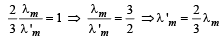31 Year NEET Previous Year Questions: Thermal Properties of Matter - Question 15

A cylindrical rod having temperature T1 and T2 at its end. The rate of flow of heat is Q1 cal/sec.If all the linear dimensions are doubled keeping temperature constant, then the rate of flow of heat Q2 will be 

Detailed Solution for 31 Year NEET Previous Year Questions: Thermal Properties of Matter - Question 15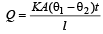Rate of heat flow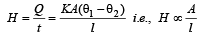Dimensions of area A = [L2], dimensions of distance l = [L]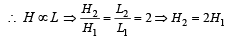31 Year NEET Previous Year Questions: Thermal Properties of Matter - Question 16

Wien's law is concerned with 

Detailed Solution for 31 Year NEET Previous Year Questions: Thermal Properties of Matter - Question 16

According to Wein's displacement law, product of wavelength belonging to maximum intensity and temperature is constant i.e.,  λmT = constant.

31 Year NEET Previous Year Questions: Thermal Properties of Matter - Question 17

Radiation from which of the following sources, approximates black body radiation best? 

Detailed Solution for 31 Year NEET Previous Year Questions: Thermal Properties of Matter - Question 17

This hole in the cavity is also called Fery's black body, it is made such that when light enters the cavity it suffers multiple reflection inside it and since with every reflection some part is absorbed so almost all the radiant light get absorbed.

31 Year NEET Previous Year Questions: Thermal Properties of Matter - Question 18

Two rods of thermal conductivities K1 and K2, cross-sections A1 and A2 and specific heats S1 and S2 are of equal lengths. The temperatures of two ends of each rod are T1 and T2. The rate of flow of heat at the steady state will be equal if 

Detailed Solution for 31 Year NEET Previous Year Questions: Thermal Properties of Matter - Question 18

Rate of heat flow for one rod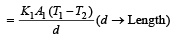.Rate of heat flow for other rod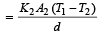In steady state,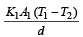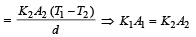31 Year NEET Previous Year Questions: Thermal Properties of Matter - Question 19

Consider a compound slab consisting of two different materials having equal thicknesses and thermal conductivities K and 2K, respectively.
The equivalent thermal conductivity of the slab is                  

Detailed Solution for 31 Year NEET Previous Year Questions: Thermal Properties of Matter - Question 19

In series, equivalent thermal conductivity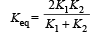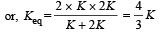31 Year NEET Previous Year Questions: Thermal Properties of Matter - Question 20

If λm denotes the wavelength at which the radiative emission from a black body at a temperature T K is maximum, then 

Detailed Solution for 31 Year NEET Previous Year Questions: Thermal Properties of Matter - Question 20

From Wein’s displacement law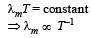31 Year NEET Previous Year Questions: Thermal Properties of Matter - Question 21

Which of the following circular rods (given radius r and length l), each made of the same material and whose ends are maintained at the same temperature will conduct most heat? 

Detailed Solution for 31 Year NEET Previous Year Questions: Thermal Properties of Matter - Question 21

We know that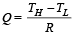Also, Thermal resistance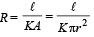Heat flow will be maximum when thermal resistance is minimum.
From given option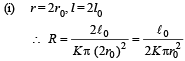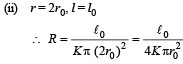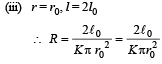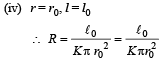It is clear that for option (2) resistance is minimum, hence, heat flow will be maximum. [Alt : (i) Rate of heat flow is directly proportional to area
(ii) inversely proportional to length.

∴ Heat flow will be maximum when r is maximum and ℓ is minimum. ]

31 Year NEET Previous Year Questions: Thermal Properties of Matter - Question 22

The temperature of in version of a thermocouple is 6200C and the neutral temperature is 3000C. What is the temperature of cold junction ? 

Detailed Solution for 31 Year NEET Previous Year Questions: Thermal Properties of Matter - Question 22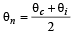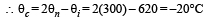31 Year NEET Previous Year Questions: Thermal Properties of Matter - Question 23

A black body at 1227°C emits radiations with maximum intensity at a wavelength of 5000Å. If the temperature of the body is increased by 1000°C, the maximum intensity will be observed at

Detailed Solution for 31 Year NEET Previous Year Questions: Thermal Properties of Matter - Question 23

Applying Wein's displacement law, λmT = constant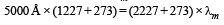= ( 5000 * 1500 ) / 2500 = 3000 Å

31 Year NEET Previous Year Questions: Thermal Properties of Matter - Question 24

A black body is at 727° C. It emits energy at a rate which is proportional to 

Detailed Solution for 31 Year NEET Previous Year Questions: Thermal Properties of Matter - Question 24

According to Stefan's law, E ∝ T4

∝ (t + 273)4 K              [727°C = (727 + 273)K]
∝ (727 + 273)4 K
∝ (1000)4 K

31 Year NEET Previous Year Questions: Thermal Properties of Matter - Question 25

If the cold junction of a thermo-couple is kept at 0°C and the hot junction is kept at T°C then the relation between neutral temperature (Tn) and temperature of inversion (Ti) is 

Detailed Solution for 31 Year NEET Previous Year Questions: Thermal Properties of Matter - Question 25

Since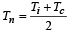= Neutral temperature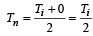[Tc = 0°C = temperature of cold junction]

31 Year NEET Previous Year Questions: Thermal Properties of Matter - Question 26

Assuming the sun to have a sph erical outer surface of radius r, radiating like a black body at temperature t°C, the power received by a unit surface, (normal to the incident rays) at a distance R from the centre of the sun is        where σ is the Stefan’s constant.

Detailed Solution for 31 Year NEET Previous Year Questions: Thermal Properties of Matter - Question 26

Power radiated by the sun at t°C

= σ(t + 273)44πr2

Power received by a unit surface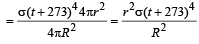31 Year NEET Previous Year Questions: Thermal Properties of Matter - Question 27

On a new scale of temperature (which is linear) and called the W scale, the freezing and boiling points of water are 39° W and 239° W respectively. What will be the temperature on the new scale, corresponding to a temperature of 39° C on the Celsius scale? 

Detailed Solution for 31 Year NEET Previous Year Questions: Thermal Properties of Matter - Question 27

For different temperature scales, we have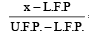= constant

Where L.F.P ⇒  Lower Fixed point
U.H.F. ⇒  Upper fixed point
where x is the measurement at that scale.
Here, if C and W be the measurements on Celsius and W scale then,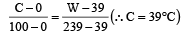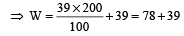= 117° W

31 Year NEET Previous Year Questions: Thermal Properties of Matter - Question 28

An electric kettle takes 4A current at 220 V. How much time will it take to boil 1 kg of water from temperature 20° C? The temperature of boiling water is 100° C. 

Detailed Solution for 31 Year NEET Previous Year Questions: Thermal Properties of Matter - Question 28

Heat required to raise the temperature of 1kg water from 20°C to 100°C is given by Q = msΔθ = (1× 4200 × 80) J
Power of kettle (P) = VI = (220 × 4)W

∴ Time taken =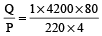= 381.81 sec = 6.36 min

31 Year NEET Previous Year Questions: Thermal Properties of Matter - Question 29

The two ends of a rod of  length L and a uniform cross-sectional area A are kept at two temperatures T1 and T2 (T1 > T2). The rate of heat transfer,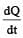through the rod in a steady state is given by: 

Detailed Solution for 31 Year NEET Previous Year Questions: Thermal Properties of Matter - Question 29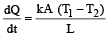[(T1–T2) is the temperature difference]

31 Year NEET Previous Year Questions: Thermal Properties of Matter - Question 30

A black body at 227°C radiates heat at the r ate of 7 cals/cm2s. At a temperature  of 727°C, the rate of heat radiated in the same units will be:

Detailed Solution for 31 Year NEET Previous Year Questions: Thermal Properties of Matter - Question 30

Accordin g to Stefan’s law E = σT4,
T1 = 500 K
T2 = 1000 K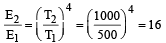∴     E2 = 16 × 7 = 112 cal / cm2s

31 Year NEET Previous Year Questions: Thermal Properties of Matter - Question 31

A cylindrical metallic rod in therrnal contact with two reservoirs of heat at its two ends conducts an amount of heat Q in time t. The metallic rod is melted and the material is formed into a rod of half the radius of the original rod. What is the amount of heat conducted by the new rod, when placed in thermal contact with the two reservoirs in time t? 

Detailed Solution for 31 Year NEET Previous Year Questions: Thermal Properties of Matter - Question 31

The rate of heat flow is given by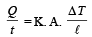Area of Original rod A = πR2 ;

Areal of new rod A' =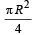Volume of original rod will be equal to the volume of new rod.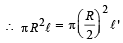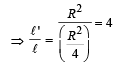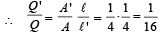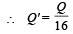31 Year NEET Previous Year Questions: Thermal Properties of Matter - Question 32

The total radiant energy per unit area, normal to the direction of incidence, received at a distance R from the centre of a star of radius r, whose outer surface radiates as a black body at a temperature T K is given by: 

Detailed Solution for 31 Year NEET Previous Year Questions: Thermal Properties of Matter - Question 32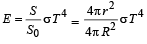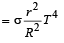31 Year NEET Previous Year Questions: Thermal Properties of Matter - Question 33

If the radius of a star is R and it acts as a black body, what would be the temperature of the star, in which the rate of energy production is Q ?  (σ stands for Stefan’S constant)

Detailed Solution for 31 Year NEET Previous Year Questions: Thermal Properties of Matter - Question 33

Stefan’s law for black body radiation Q = σe AT4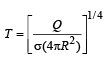Here e = 1
A = 4πR2

31 Year NEET Previous Year Questions: Thermal Properties of Matter - Question 34

Liquid oxygen at 50 K is heated to 300 K at constant pressure of 1 atm. The rate of heating is constant. Which one of the following graphs represents the variation of temperature with time? 

Detailed Solution for 31 Year NEET Previous Year Questions: Thermal Properties of Matter - Question 34

Initially liquid oxygen will gain the temperature up to its boiling temperature then it change its state to gas. After this again its temperature will increase, so corresponding graph will be

31 Year NEET Previous Year Questions: Thermal Properties of Matter - Question 35

A slab of stone of area 0.36 m2 and thickness 0.1 m is exposed on the lower surface to steam at 100°C. A block of ice at 0°C rests on the upper surface of the slab. In one hour 4.8 kg of ice is melted. The thermal conductivity of slab is : (Given latent heat of fusion of ice = 3.36 × 105 Jkg–1.) : [2012M]

Detailed Solution for 31 Year NEET Previous Year Questions: Thermal Properties of Matter - Question 35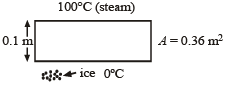Rate of heat given by steam = Rate of heat taken by ice where
K = Thermal conductivity of the slab
m = Mass of the ice
L = Latent heat of melting/fusion
A = Area of the slab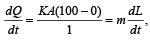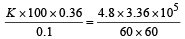K =1.24 J/m/s/°C

31 Year NEET Previous Year Questions: Thermal Properties of Matter - Question 36

A piece of iron is heated in a flame. It first becomes dull red then becomes reddish yellow and finally turns to white hot. The correct explanation for the above observation is possible by using [NEET 2013]

Detailed Solution for 31 Year NEET Previous Year Questions: Thermal Properties of Matter - Question 36

Wein’s displacement law According to this law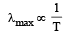or,  λmax × T = constant
So, as the temperature increases λ decreases.

31 Year NEET Previous Year Questions: Thermal Properties of Matter - Question 37

The molar specific heats of an ideal gas at constant pressure and volume are denoted by Cp and Cv, respectively. If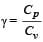and R is theuniversal gas constant, then Cv is equal to [NEET 2013]

Detailed Solution for 31 Year NEET Previous Year Questions: Thermal Properties of Matter - Question 37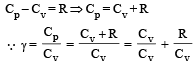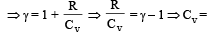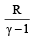31 Year NEET Previous Year Questions: Thermal Properties of Matter - Question 38

The density of water at 20°C is 998 kg/m3 and at 40°C 992 kg/m3. The coefficient of volume expansion of water is [NEET Kar. 2013]

Detailed Solution for 31 Year NEET Previous Year Questions: Thermal Properties of Matter - Question 38

From question, Δρ = (998 – 992) kg/m3 = 6 kg/m3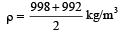= 995 kg/m3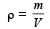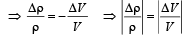∴ Coefficient of volume expansion of water,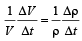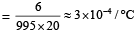31 Year NEET Previous Year Questions: Thermal Properties of Matter - Question 39

Two metal rods 1 and 2 of same lengths have same temperature difference between their ends.Their thermal conductivities are K1 and K2 and cross sectional areas A1 and A2, respectively. If the rate of heat conduction in rod 1 is four times that in rod 2, then [NEET Kar. 2013]

Detailed Solution for 31 Year NEET Previous Year Questions: Thermal Properties of Matter - Question 39

Q1 = 4Q2 (Given)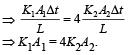## Physics Class 11

127 videos|464 docs|210 tests
 Use Code STAYHOME200 and get INR 200 additional OFF Use Coupon Code
Information about 31 Year NEET Previous Year Questions: Thermal Properties of Matter Page
In this test you can find the Exam questions for 31 Year NEET Previous Year Questions: Thermal Properties of Matter solved & explained in the simplest way possible. Besides giving Questions and answers for 31 Year NEET Previous Year Questions: Thermal Properties of Matter, EduRev gives you an ample number of Online tests for practice

## Physics Class 11

127 videos|464 docs|210 tests

### How to Prepare for NEET

Read our guide to prepare for NEET which is created by Toppers & the best Teachers